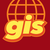# Calculating Cumulative Field Values in ArcMap using a case field

1908
3
05-09-2020 05:42 AMNew Contributor II

I want to calculate cumulative field values using an case field from another column in ArcGIS 10.4.

For example, if I have an "ID" column and "Count" column inside my attribute table, I want to calculate cumulative values for each year separately.

I have used this:

I can do that if I select all rows with the same ID and run the field calculator, but that is a lot of manual work, so I want to use "ID" field as case field.

What is the best way to do that?

Tags (4)
1 Solution

Accepted SolutionsbyMVP Frequent Contributor
``````count = 0
id_list =[]
def cummulativeCount(increment, id_row_value):
global count
global id_list

id_list.append(id_row_value)

if len(id_list)>=2 and id_row_value == id_list[-2]:
count += int(increment)

elif len(id_list)<2:
count += int(increment)

else:
count = int(increment)

return count‍‍‍‍‍‍‍‍‍‍‍‍‍‍‍‍‍‍‍‍‍‍``````

cummulativeCount( !count_! , !id! )

3 RepliesbyMVP Frequent Contributor
``````count = 0
id_list =[]
def cummulativeCount(increment, id_row_value):
global count
global id_list

id_list.append(id_row_value)

if len(id_list)>=2 and id_row_value == id_list[-2]:
count += int(increment)

elif len(id_list)<2:
count += int(increment)

else:
count = int(increment)

return count‍‍‍‍‍‍‍‍‍‍‍‍‍‍‍‍‍‍‍‍‍‍``````

cummulativeCount( !count_! , !id! )New Contributor II

Thank you very much!byMVP Frequent Contributor

нема на чему